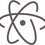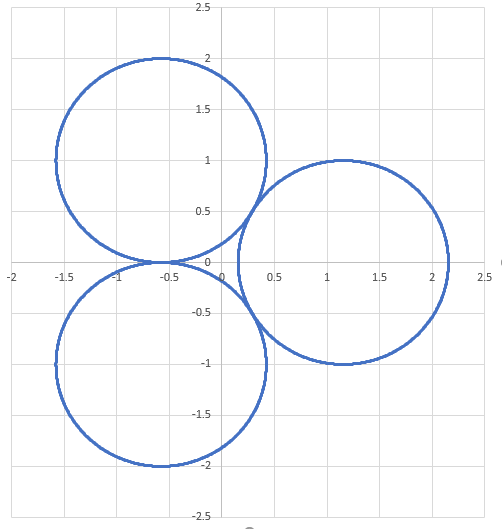# Fidget spinner

What is the moment of inertia of a fidget spinner assuming mass of the spinner as M and radius of the disc end as $R$? (Assuming that the Fidget spinner is made of three discs )4 years ago

This discussion board is a place to discuss our Daily Challenges and the math and science related to those challenges. Explanations are more than just a solution — they should explain the steps and thinking strategies that you used to obtain the solution. Comments should further the discussion of math and science.

When posting on Brilliant:

• Use the emojis to react to an explanation, whether you're congratulating a job well done , or just really confused .
• Ask specific questions about the challenge or the steps in somebody's explanation. Well-posed questions can add a lot to the discussion, but posting "I don't understand!" doesn't help anyone.
• Try to contribute something new to the discussion, whether it is an extension, generalization or other idea related to the challenge.

MarkdownAppears as
*italics* or _italics_ italics
**bold** or __bold__ bold
- bulleted- list
• bulleted
• list
1. numbered2. list
1. numbered
2. list
Note: you must add a full line of space before and after lists for them to show up correctly
paragraph 1paragraph 2

paragraph 1

paragraph 2

[example link](https://brilliant.org)example link
> This is a quote
This is a quote
    # I indented these lines
# 4 spaces, and now they show
# up as a code block.

print "hello world"
# I indented these lines
# 4 spaces, and now they show
# up as a code block.

print "hello world"
MathAppears as
Remember to wrap math in $$ ... $$ or $ ... $ to ensure proper formatting.
2 \times 3 $2 \times 3$
2^{34} $2^{34}$
a_{i-1} $a_{i-1}$
\frac{2}{3} $\frac{2}{3}$
\sqrt{2} $\sqrt{2}$
\sum_{i=1}^3 $\sum_{i=1}^3$
\sin \theta $\sin \theta$
\boxed{123} $\boxed{123}$

Sort by:

How exactly do you want it to be modeled?

- 4 years ago

it should consits of only three discs of each radius R and mass of each disc as M/3

- 4 years ago

Do the disks have holes in them? If so, what are the inner and outer radii. And how are the disks connected to each other? If you look at an actual fidget spinner, there is a complicated connection between them. We'll probably need to simplify the connection.

- 4 years ago

there are no holes and the discs are stuck together using an adhesive.

- 4 years ago

I have an answer. This is a pretty nice problem. If you have the answer, perhaps you could post it as a problem.

- 4 years ago

- 3 years, 12 months agoModel the fidget spinner as three disks with their centers as the vertices of an equilateral triangle. The disk radius is $R$ and the distance ($d$) of each disk center to the origin is $\frac{2}{\sqrt{3}} R$.

The moment of a disk of mass $M$ about it's center is $\frac{M R^2}{2}$. Since all three disks are the same distance from the origin, consider the total mass to be concentrated in one of them. We must use the Parallel Axis Theorem to calculate the moment with respect to the origin.

$I = \frac{M R^2}{2} + Md^2 = \frac{M R^2}{2} + \frac{4}{3} MR^2 = \boxed{\frac{11}{6} MR^2}$

- 3 years, 12 months ago

Actually, there is also one disc with centre at the origin.

- 3 years, 12 months ago

In that case it's easier. $\frac{25}{2} M R^2$

- 3 years, 12 months ago

so added up all moment of inertia of all the discs along the axis at origin right?

- 3 years, 12 months ago

Yes, that's right. If you agree with my answer, you can post it. Thanks

- 3 years, 12 months ago

In that case, how about posting this as a question ?

- 3 years, 12 months ago